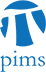## Small solutions of Nonlinear Schrodinger Equations with Many Bound States

• Date: 12/01/2009
Lecturer(s):
Tuoc V. Phan (UBC)
Location:

University of British Columbia

Description:

Consider a nonlinear Schr\"{o}dinger equation in $\mathbb{R}^3$ with a short-range potential. The linear Hamiltonian is assumed to have three or more eigenvalues satisfying some resonance conditions. We study the asymptotic behavior at time infinity of solutions with small initial data in $H^1 \cap L^1(\mathbb{R}^3)$. The results include the case that all of the eigenvalues are simple and also the case that the second eigenvalues are degenerate. These are joint works with Stephen Gustafson, Kenji Nakanishi and Tai-Peng Tsai.

Schedule:

3:30pm - 4:30pm, WMAX 110.